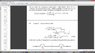# Klein-Gordon Propagator

guillefix
Hello, I'm looking at the following computation from the Peskin and Schroeder's book:

See file attached

In the second page, the second term that's being integrated, I don't understand why it has a negative i in the exponential, that'll keep the energy term the same, but will swap the sign of the spatial terms, wouldn't it?

Thank you beforehand

#### Attachments

•Qft.jpg
32.7 KB · Views: 538

Lapidus
Below equation 2.53, they say when x-y is spacelike, a Lorentz transformation can take (x-y) to -(x-y).

First of all, it's important to keep in mind that $x^0>y^0$ is assumed, because otherwise the equation would be wrong, because (2.54) with the contour given is the retarded propagator, i.e.,
$$\mathrm{i} G_{\text{ret}}(x,y)=\Theta(x^0-y^0) \langle 0 |[\phi(x),\phi(y)]|0 \rangle.$$
Further, the minus sign in the second term of (2.54) is correct, and the handwritten "correction" wrong. You get this from closing the contour in the lower complex $p^0$ half-plane. You must close the contour there in order to make this additional part go away when carrying it to infinity, and that's why you get the commutator with this contour only if $x^0>y^0$. You come back to the original expression you started with by substituting $\vec{p}'=-\vec{p}$ in this second term.
For space-like separations you get 0 as it must be, because for space-like separations of $x$ and $y$ you can find a frame of reference, where $x^0=y^0$ and there according to the canonical equal-time commutation relations the fields commute. Since the commutator function is a scalar function, it vanishes thus for all space-like separated arguments. This should be so because of the demand of microcausality for local observables.
•